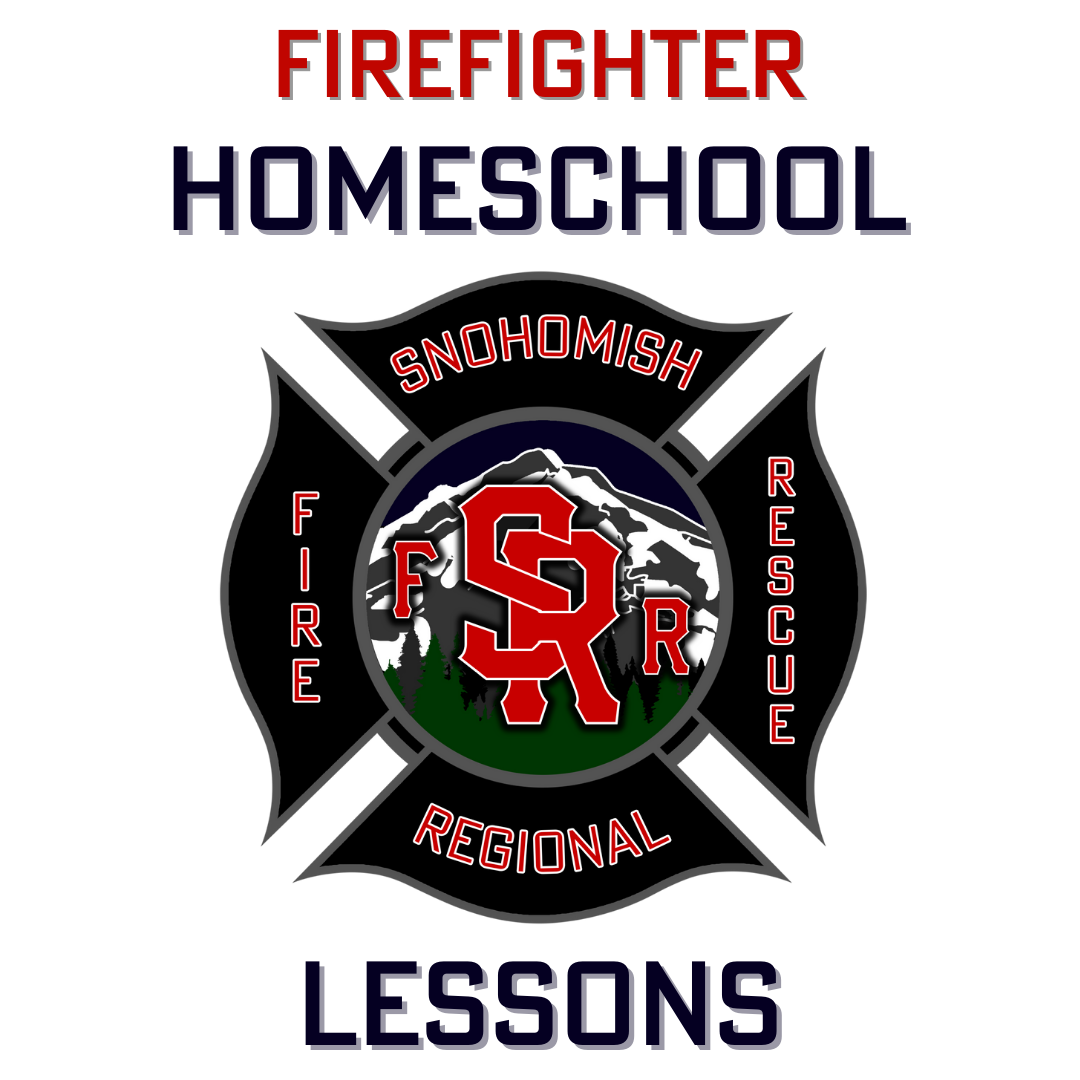### Firefighter Homeschool Lesson Details• This lesson reviews addition, subtraction, multiplication, and measurement concepts as it relates to fighting fires. Also join us on a virtual tour of a fire engine as one of our firefighters discusses how math applies to fighting a fire!
• This lesson aligns with the following standard(s):
• Common Core State Standards:
• CCSS.MATH.CONTENT.2.OA.A.1
• CCSS.MATH.CONTENT.2.MD.A.1
• CCSS.MATH.CONTENT.2.MD.A.4
• Common Core State Standards:
• CCSS.MATH.CONTENT.2.OA.A.1
Use addition and subtraction within 100 to solve one- and two-step word problems involving situations of adding to, taking from, putting together, taking apart, and comparing, with unknowns in all positions, e.g., by using drawings and equations with a symbol for the unknown number to represent the problem.
• CCSS.MATH.CONTENT.2.MD.A.1
Measure the length of an object by selecting and using appropriate tools such as rulers, yardsticks, meter sticks, and measuring tapes.
• CCSS.MATH.CONTENT.2.MD.A.4
Measure to determine how much longer one object is than another, expressing the length difference in terms of a standard length unit.